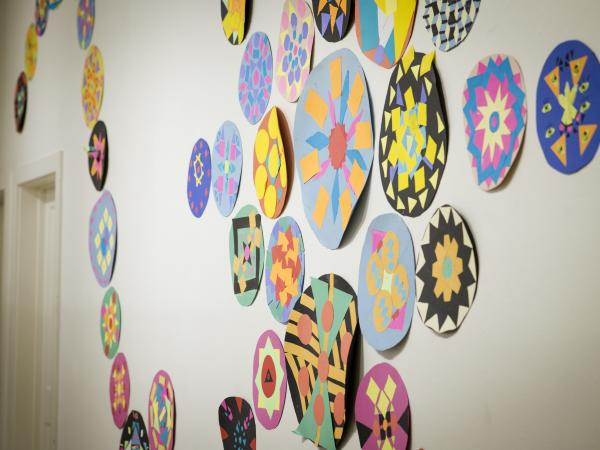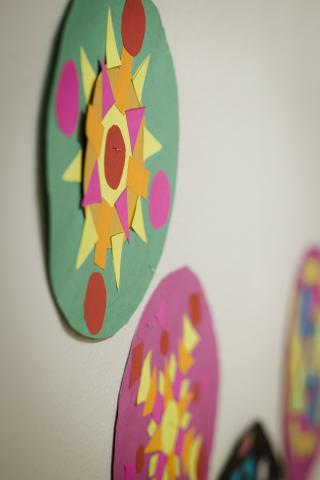# Math Mandalas—Geometry

March 2, 2020
Image### Students explore geometry concepts through their design and creation of mandalas.

They will use their knowledge of circumference and area to develop a circle which will then become the container for their mandala designs and create their mandala by calculating the area and perimeter of different sized triangles and squares. Key concepts surrounding mandala design will be explored, including pattern, repetition, symmetry, proportion, and shape.

### Objectives:

• Students will use formulas for area and circumference of a circle to develop a mandala
• Students will demonstrate understanding of the principles of symmetry, repetition, pattern, and shape through the design of their mandala
• Students will use at least three different sized shapes and calculate the area of each
• Students will choose at least one shape to create two different scales
• Students will write a two-to-three-sentence statement about their use of different formulas to construct their mandala

### Basic Lesson Outline:

• Introduce the project
• Show a mandala in progress, explaining how the circle was calculated
• Students begin working on their mandalas
• Students continue working on mandalas
• Students continue and complete mandalas
• Students present and display their mandalas

### Art Supplies:

• Poster board (multiple colors)
• Rulers
• Scissors
• Glue
• Pencils
• String

### Other Resources:

• Examples of mandalas created
• Slide show to introduce project and related artwork (see outline at the end of the lesson plan)### Idaho State Learning Standards:

Arts and Humanities: Anchor Standard 4: Convey meaning through the presentation/performance/production of an original work or unique interpretation of a work

• Objective PR1.1 Combine knowledge and understanding from two or more disciplines to present/perform their original or interpreted works for an audience
• Objective PR1.2 Convey meaning through their presentation/performance

CCSS.MATH.CONTENT.7.G.A.1
Solve problems involving scale drawings of geometric figures, including computing actual lengths and areas from a scale drawing and reproducing a scale drawing at a different scale.

CCSS.MATH.CONTENT.7.G.B.4
Know the formulas for the area and circumference of a circle and use them to solve problems; give an informal derivation of the relationship between the circumference and area of a circle.

CCSS.MATH.CONTENT.7.G.B.6
Solve real-world and mathematical problems involving area, volume and surface area of two- and three-dimensional objects composed of triangles, quadrilaterals, polygons, cubes, and right prisms.

• Subject area language: circumference, area, diameter, radius, and perimeter
• Art language: mandala, symmetry, repetition, pattern, shape, and mindfulness

### Student Use of Vocabulary:

Students will use the words when creating their projects, when writing about their projects, and when discussing their projects

### Student Grouping:

Students will work independently